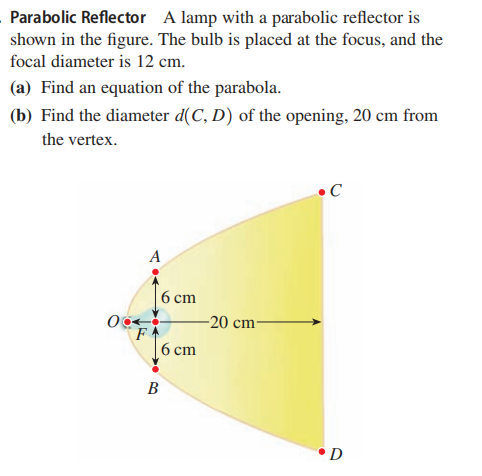### ¿Todavía tienes preguntas de matemáticas?

Pregunte a nuestros tutores expertos
Algebra
PreguntaA lamp with a parabolic reflector is shown in the figure. The bulb is placed at the focus, and the focal diameter is $$12 cm$$ .

(a) Find an equation of the parabola.

(b) Find the diameter $$d ( C , D )$$ of the opening, $$20 cm$$ from the vertex.

$$\text { (a) } y ^ { 2 } = 12 x \quad \text { (b) } 8 \sqrt { 15 } \approx 31 cm$$